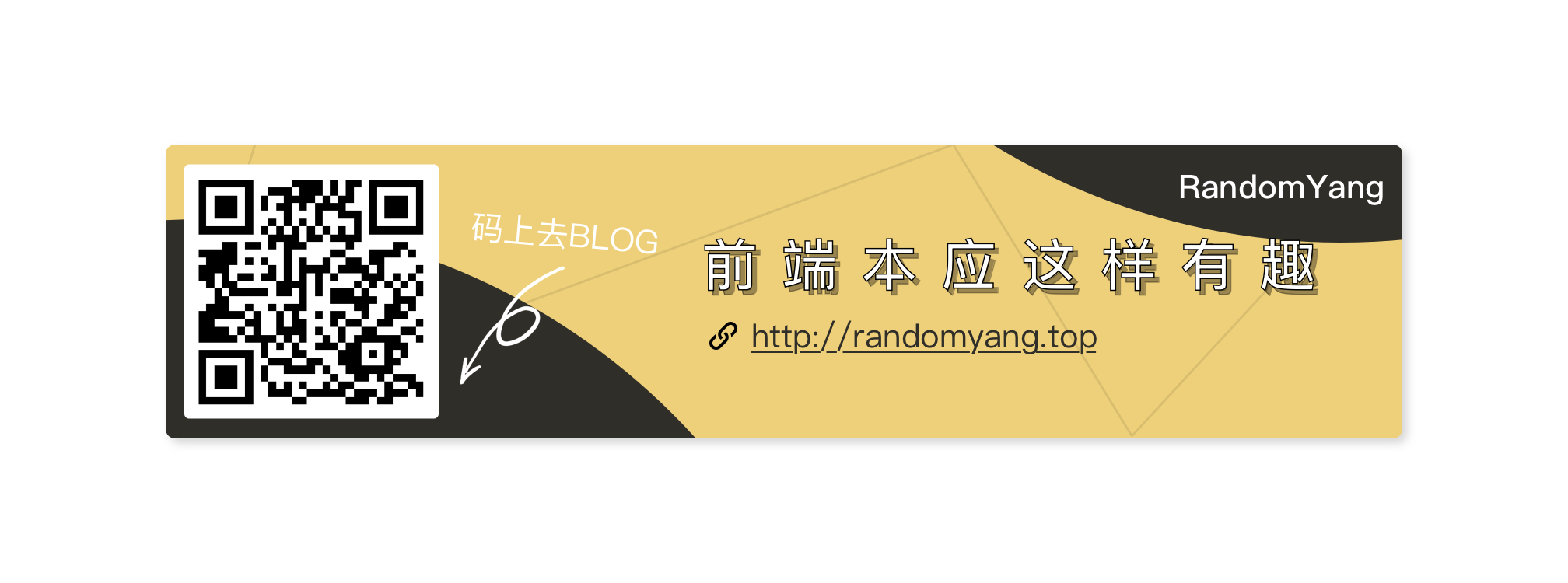# RandomYang

Code + Design => Magic

[译]使用Three.js制作有粘稠感的图像悬停效果

# [译]使用Three.js制作有粘稠感的图像悬停效果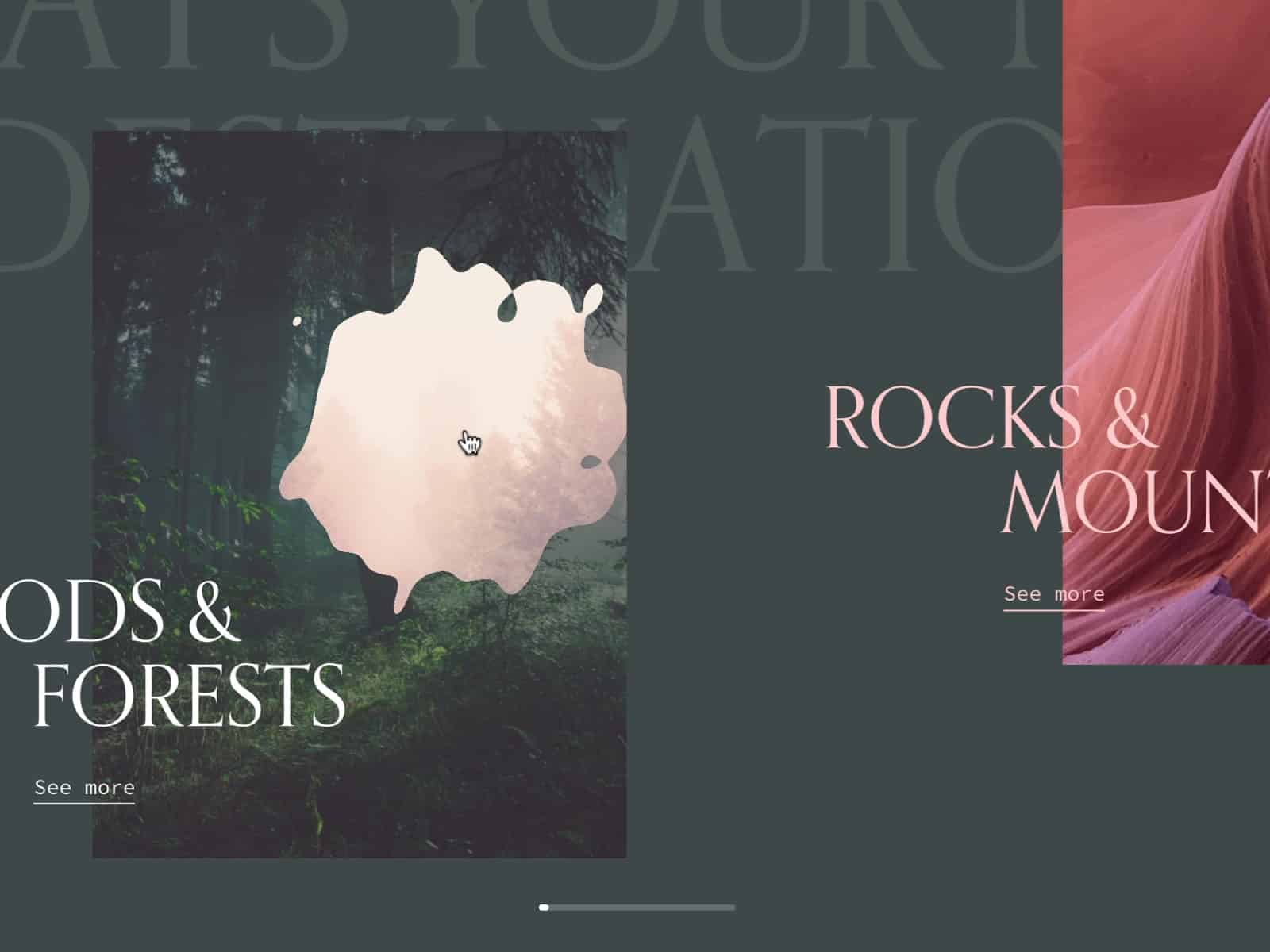## 在 DOM 中创建场景(scene)

// index.html

<section class="container">
<article class="tile">
<figure class="tile__figure">
<img data-src="path/to/my/image.jpg" data-hover="path/to/my/hover-image.jpg" class="tile__image" alt="My image" width="400" height="300" />
</figure>
</article>
</section>

<canvas id="stage"></canvas>
// style.css

.container {
display: flex;
align-items: center;
justify-content: center;
width: 100%;
height: 100vh;
z-index: 10;
}

.tile {
width: 35vw;
flex: 0 0 auto;
}

.tile__image {
width: 100%;
height: 100%;
object-fit: cover;
object-position: center;
}

canvas {
position: fixed;
left: 0;
top: 0;
width: 100%;
height: 100vh;
z-index: 9;
}## 在 JavaScript 中创建场景(scene)

// Scene.js

import * as THREE from 'three'

export default class Scene {
constructor() {
this.container = document.getElementById('stage')

this.scene = new THREE.Scene()
this.renderer = new THREE.WebGLRenderer({
canvas: this.container,
alpha: true,
})

this.renderer.setSize(window.innerWidth, window.innerHeight)
this.renderer.setPixelRatio(window.devicePixelRatio)

this.initLights()
}

initLights() {
const ambientlight = new THREE.AmbientLight(0xffffff, 2)
}
}

// Scene.js

const perspective = 800

constructor() {
// ...
this.initCamera()
}

initCamera() {
const fov = (180 * (2 * Math.atan(window.innerHeight / 2 / perspective))) / Math.PI

this.camera = new THREE.PerspectiveCamera(fov, window.innerWidth / window.innerHeight, 1, 1000)
this.camera.position.set(0, 0, perspective)
}

// Scene.js

constructor() {
// ...
this.update()
}

update() {
requestAnimationFrame(this.update.bind(this))

this.renderer.render(this.scene, this.camera)
}

## 用正确的尺寸创建平面

// Scene.js

import Figure from './Figure'

constructor() {
// ...
this.figure = new Figure(this.scene)
}
// Figure.js

export default class Figure {
constructor(scene) {
this.$image = document.querySelector('.tile__image') this.scene = scene this.loader = new THREE.TextureLoader() this.image = this.loader.load(this.$image.dataset.src)
this.hoverImage = this.loader.load(this.$image.dataset.hover) this.sizes = new THREE.Vector2(0, 0) this.offset = new THREE.Vector2(0, 0) this.getSizes() this.createMesh() } } 首先，我们创建另一个类，将场景作为属性传递给该类。我们设置了两个新的矢量，尺寸和偏移，用于存储DOM图像的尺寸和位置。 此外，我们将使用TextureLoader来“加载”图像并将其转换为纹理。我们需要这样做，因为我们想在着色器中使用这些图片。 我们需要在类中创建一个方法来处理图像的加载并等待回调。我们可以使用异步功能来实现这一目标，但对于本教程而言，我们将其保持简单。请记住，您可能需要出于自身目的对它进行一些重构。 // Figure.js // ... getSizes() { const { width, height, top, left } = this.$image.getBoundingClientRect()

this.sizes.set(width, height)
this.offset.set(left - window.innerWidth / 2 + width / 2, -(top - window.innerHeight / 2 + height / 2))
}
// ...

// Figure.js

// ...
createMesh() {
this.geometry = new THREE.PlaneBufferGeometry(1, 1, 1, 1)
this.material = new THREE.MeshBasicMaterial({
map: this.image
})

this.mesh = new THREE.Mesh(this.geometry, this.material)

this.mesh.position.set(this.offset.x, this.offset.y, 0)
this.mesh.scale.set(this.sizes.x, this.sizes.y, 1)

}
// ...

## 获取鼠标坐标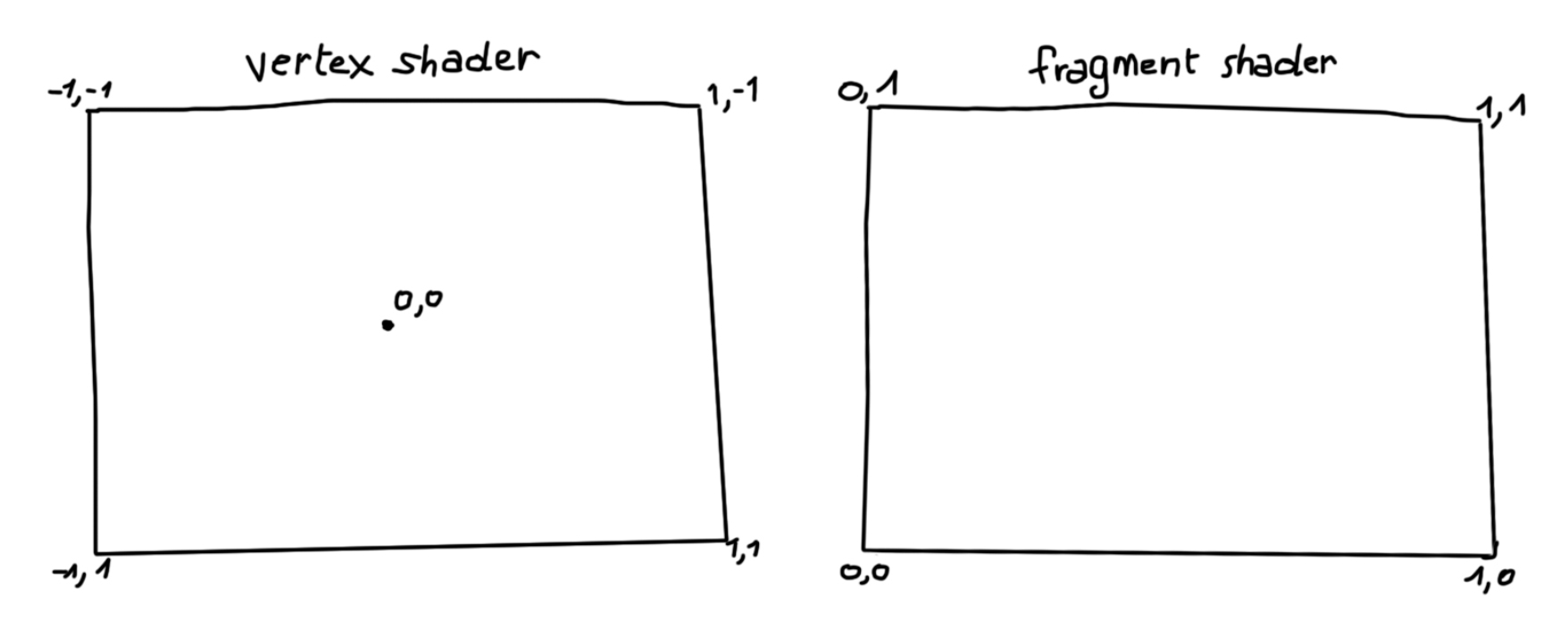// Figure.js

// ...

this.mouse = new THREE.Vector2(0, 0)
window.addEventListener('mousemove', (ev) => { this.onMouseMove(ev) })

// ...

onMouseMove(event) {
TweenMax.to(this.mouse, 0.5, {
x: (event.clientX / window.innerWidth) * 2 - 1,
y: -(event.clientY / window.innerHeight) * 2 + 1,
})

TweenMax.to(this.mesh.rotation, 0.5, {
x: -this.mouse.y * 0.3,
y: this.mouse.x * (Math.PI / 6)
})
}// Figure.js

// ...

this.uniforms = {
u_image: { type: 't', value: this.image },
u_imagehover: { type: 't', value: this.hover },
u_mouse: { value: this.mouse },
u_time: { value: 0 },
u_res: { value: new THREE.Vector2(window.innerWidth, window.innerHeight) }
}

uniforms: this.uniforms,
})

update() {
this.uniforms.u_time.value += 0.01
}

## 技巧背后的原理及如何使用噪声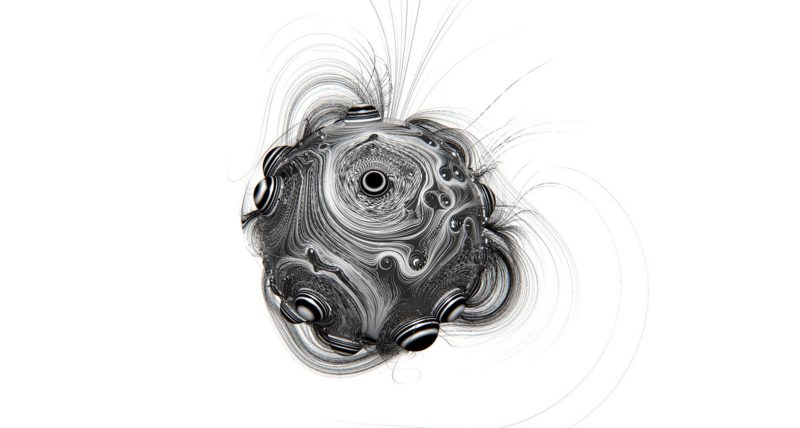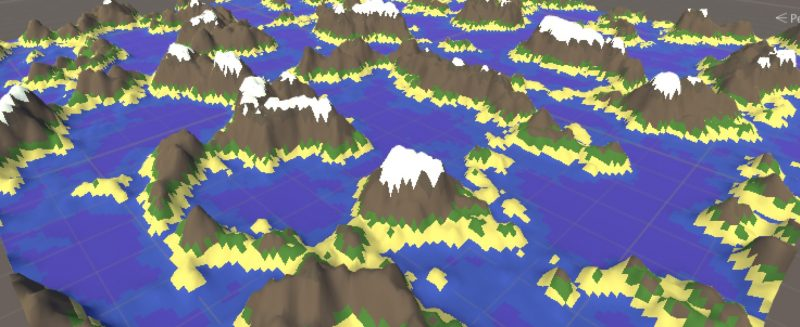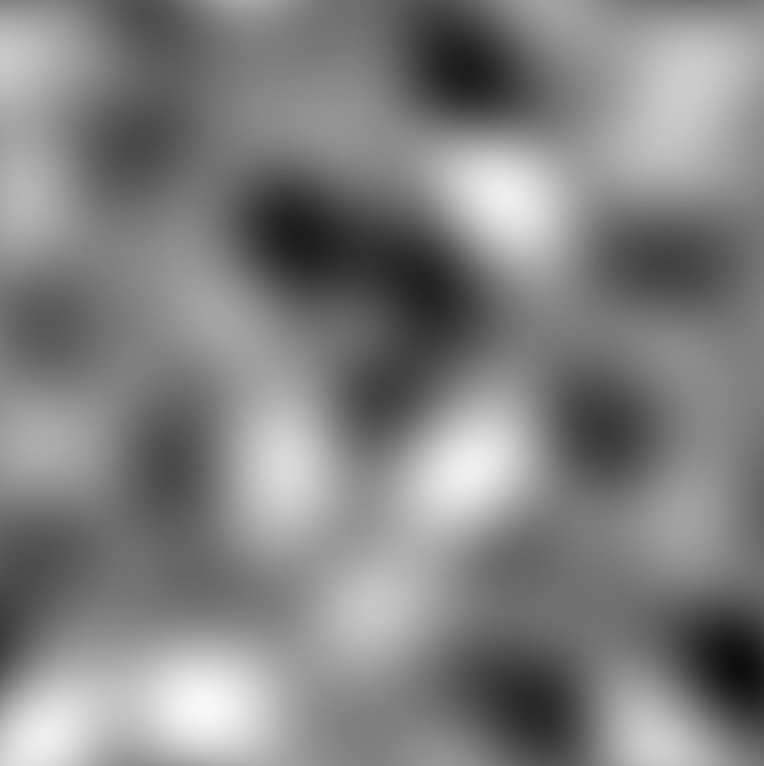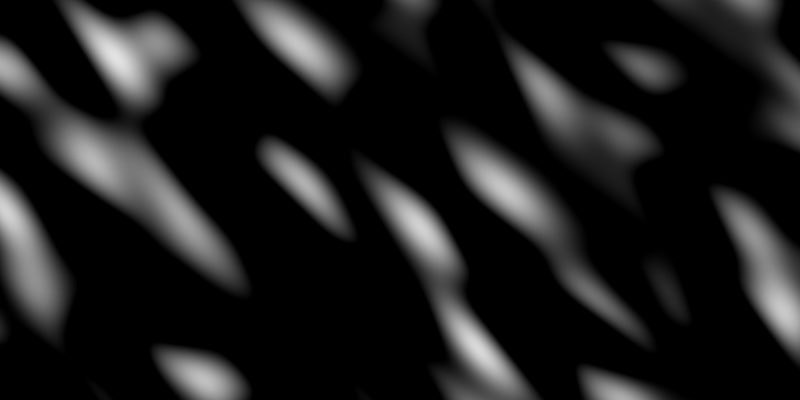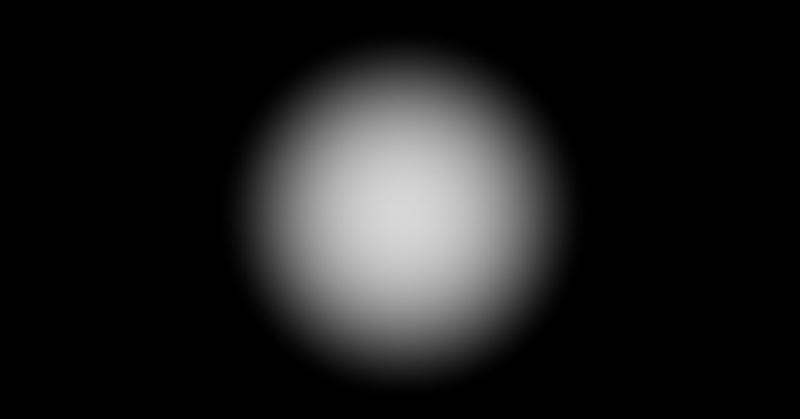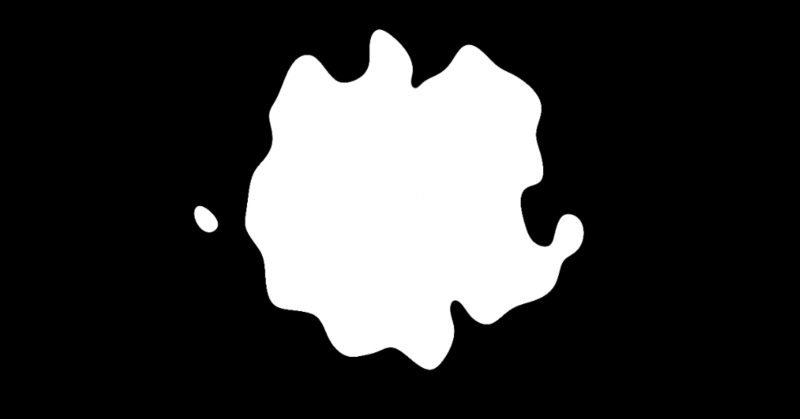## 着色器

 // vertexShader.glsl
varying vec2 v_uv;

void main() {
v_uv = uv;

gl_Position = projectionMatrix * modelViewMatrix * vec4(position, 1.0);
}

• 位置（vec3）：网格每个顶点的坐标
• uv（vec2）：纹理的坐标
• 法线（vec3）：网格中每个顶点的法线。

## 创建圆形

// fragmentShader.glsl
uniform vec2 u_mouse;
uniform vec2 u_res;

float circle(in vec2 _st, in float _radius, in float blurriness){
vec2 dist = _st;
}

void main() {
vec2 st = gl_FragCoord.xy / u_res.xy - vec2(1.);
// tip: use the following formula to keep the good ratio of your coordinates
st.y *= u_res.y / u_res.x;

vec2 mouse = u_mouse;
// tip2: do the same for your mouse
mouse.y *= u_res.y / u_res.x;
mouse *= -1.;

vec2 circlePos = st + mouse;
float c = circle(circlePos, .03, 2.);

gl_FragColor = vec4(vec3(c), 1.);
}## 创建一些噪噪噪噪声声声～～

// fragmentShader.glsl
#pragma glslify: snoise2 = require('glsl-noise/simplex/2d')

//...

varying vec2 v_uv;

uniform float u_time;

void main() {
// ...

float n = snoise2(vec2(v_uv.x, v_uv.y));

gl_FragColor = vec4(vec3(n), 1.);
}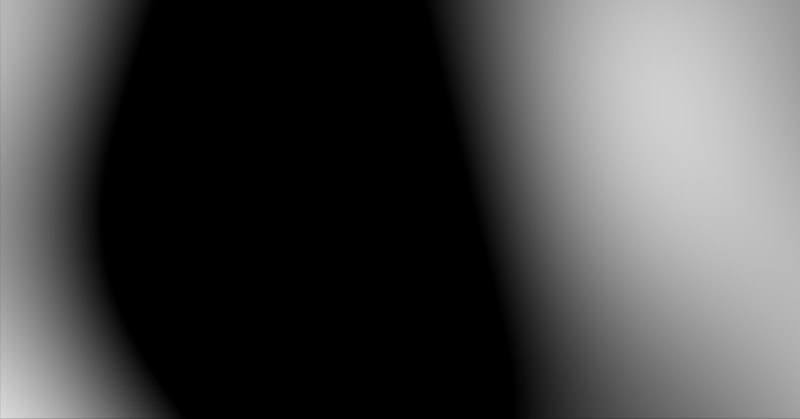// fragmentShader.glsl

float offx = v_uv.x + sin(v_uv.y + u_time * .1);
float offy = v_uv.y - u_time * 0.1 - cos(u_time * .001) * .01;

float n = snoise2(vec2(offx, offy) * 5.) * 1.;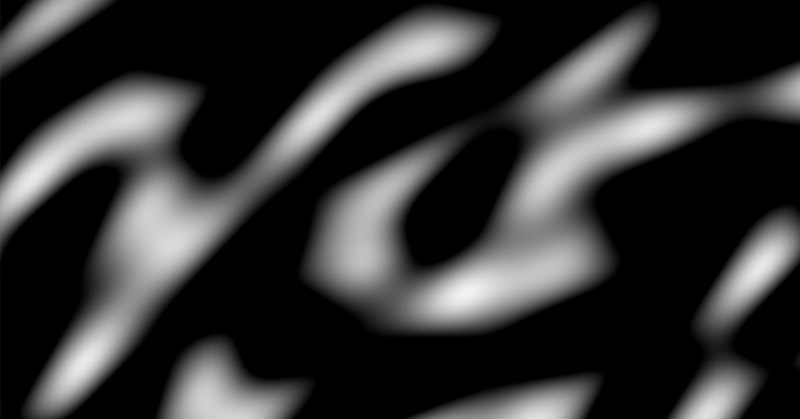float n = snoise3(vec3(offx, offy, u_time * .1) * 4.) * .5;

## 合并纹理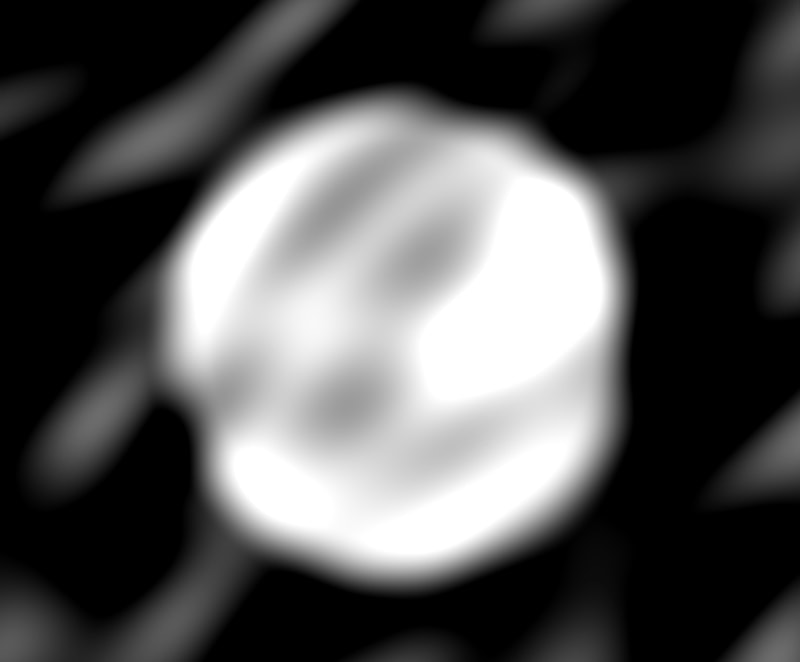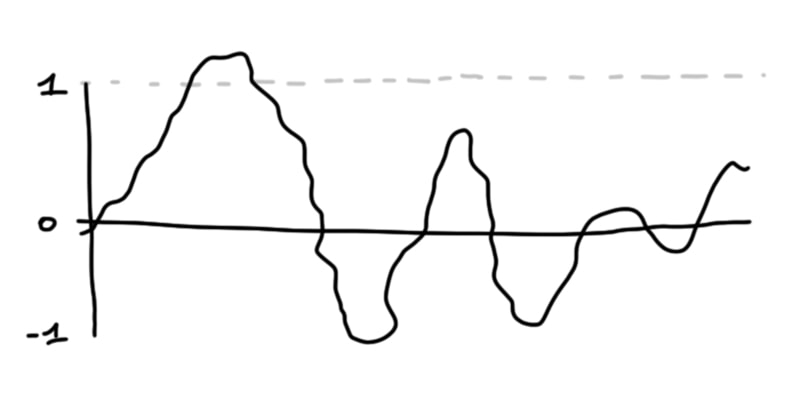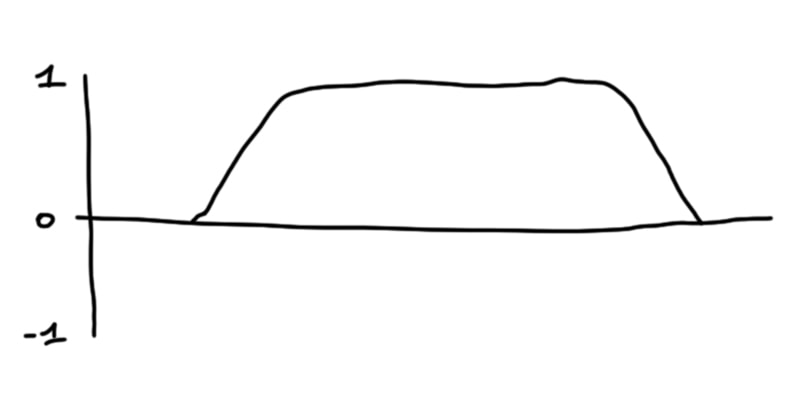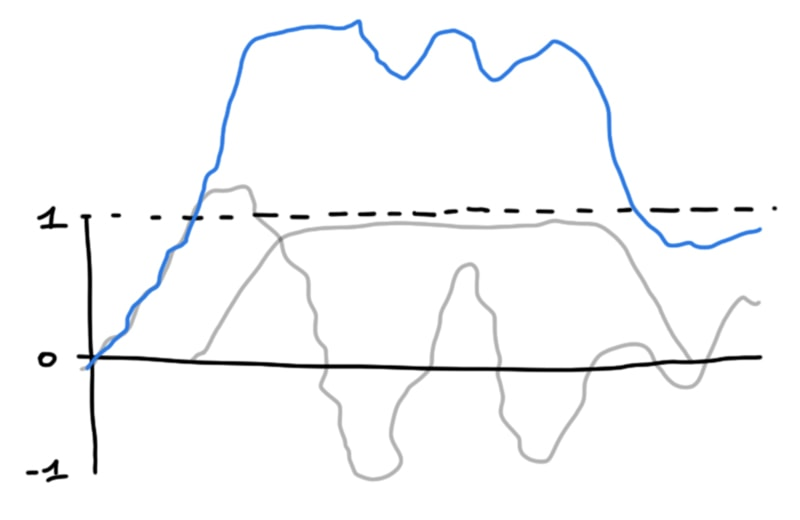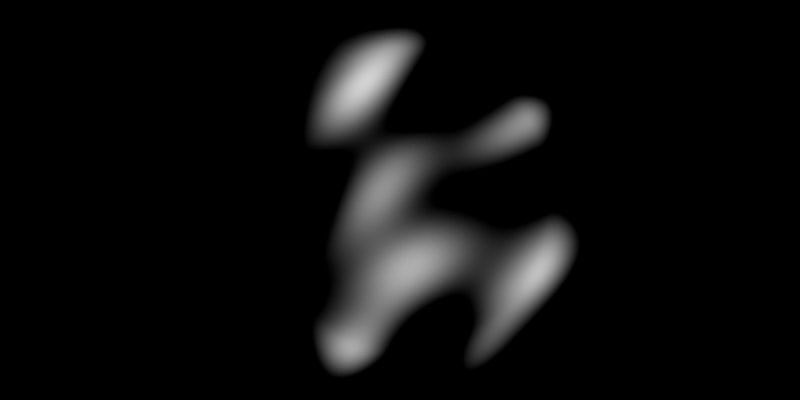float n = snoise(vec3(offx, offy, u_time * .1) * 4.) - 1.;

float c = circle(circlePos, 0.3, 0.3) * 2.5;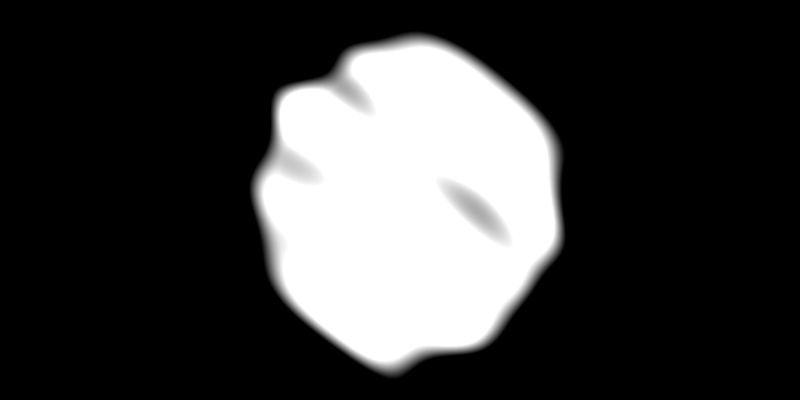float finalMask = smoothstep(0.4, 0.5, n + c);

gl_FragColor = vec4(vec3(finalMask), 1.);uniform sampler2D u_image;
uniform sampler2D u_imagehover;

// ...

vec4 image = texture2D(u_image, uv);
vec4 hover = texture2D(u_imagehover, uv);

vec4 finalImage = mix(image, hover, finalMask);

gl_FragColor = finalImage;

// ...

float c = circle(circlePos, 0.3, 2.) * 2.5;

float n = snoise3(vec3(offx, offy, u_time * .1) * 8.) - 1.;

float finalMask = smoothstep(0.4, 0.5, n + pow(c, 2.));

// ...## 参考以及感谢